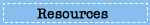# Sample Questions

## What questions are used in the NAEP mathematics assessment?

Explore sample questions from the mathematics assessment, and see how the NAEP mathematics questions relate to student performance.## Test Yourself in Mathematics

Select one of the grade tabs below to try out 10 questions from the 2009 NAEP mathematics assessment. After answering all the questions, compare your score with that of students nationally.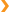Grade 4 Sample Questions

Question 1 of 10: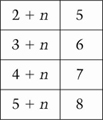What number does n represent in the table?

1. 2
2. 3
3. 4
4. 5
Question 2 of 10 :

It takes Ms. Wylie 15 minutes to drive from her house to the store. Which is the best estimate of the distance from her house to the store?

1.     5 feet
2.    5 miles
3.    20 feet
4.  200 miles
Question 3 of 10:

Kiara set her beads on a table to make a repeating pattern. Some of the beads rolled off the table.
Here is what was left.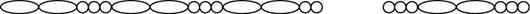Which of the following should Kiara use to replace the missing beads in the pattern?

1.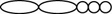2.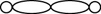3.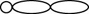4.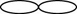Question 4 of 10: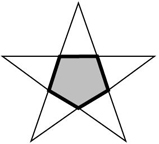What is the shape of the shaded figure inside the star?

1. Hexagon
2. Pentagon
4. Triangle

Question 5 of 10: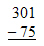1. 226
2. 235
3. 236
4. 374

Question 6 of 10:One student will be chosen at random from the list above. What is the probability that the student's name begins with the letter P?

1. 1 out of 3
2. 1 out of 10
3. 3 out of 7
4. 3 out of 10

Question 7 of 10: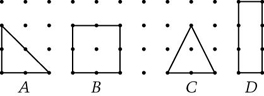Which figure has the greatest area?

1. A
2. B
3. C
4. D

Question 8 of 10:

Which picture shows that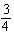is the same as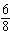?

1.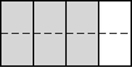2.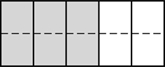3.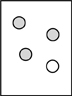4.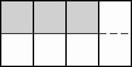Question 9 of 10:

A loaded trailer truck weighs 26,643 kilograms. When the trailer truck is empty, it weighs 10,547 kilograms. About how much does the load weigh?

1. 14,000 kilograms
2. 16,000 kilograms
3. 18,000 kilograms
4. 36,000 kilograms

Question 10 of 10:

Which fraction has a value closest to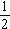?

1.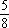2.

3.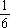4.

5.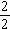6.

7.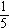The chart below shows the percentage of questions that you answered correctly, as well as how students at each of the achievement levels performed on this set of questions. For instance, fourth-grade students at Proficient had a percentage correct of 77% on average.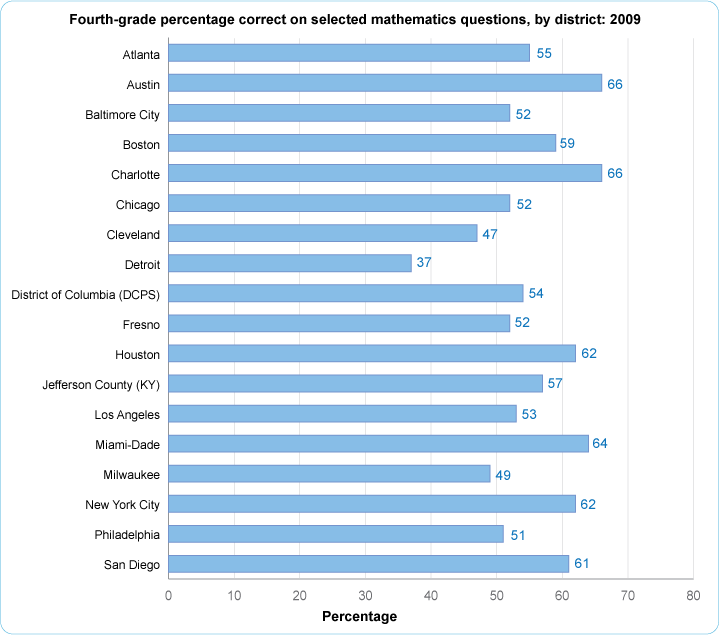•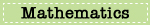•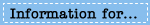•